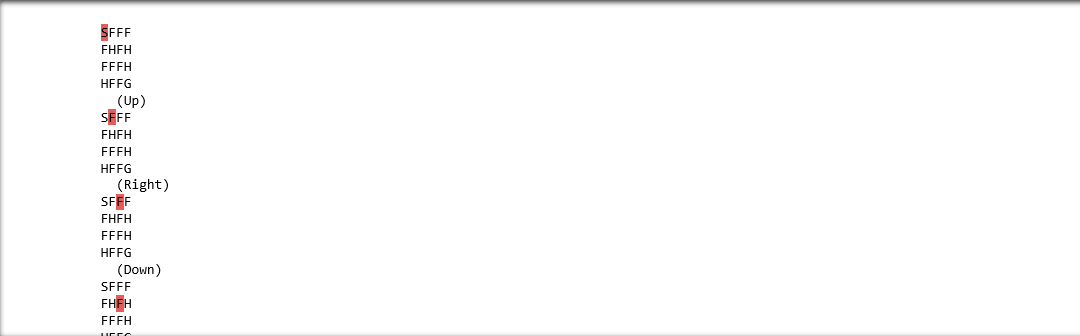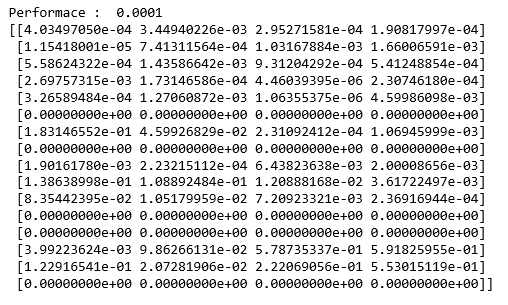GeeksforGeeks App
Open AppBrowser
Continue

# SARSA Reinforcement Learning

Prerequisites: Q-Learning technique
SARSA algorithm is a slight variation of the popular Q-Learning algorithm. For a learning agent in any Reinforcement Learning algorithm it’s policy can be of two types:-

1. On Policy: In this, the learning agent learns the value function according to the current action derived from the policy currently being used.
2. Off Policy: In this, the learning agent learns the value function according to the action derived from another policy.

Q-Learning technique is an Off Policy technique and uses the greedy approach to learn the Q-value. SARSA technique, on the other hand, is an On Policy and uses the action performed by the current policy to learn the Q-value.
This difference is visible in the difference of the update statements for each technique:-

1. Q-Learning:2. SARSA:Here, the update equation for SARSA depends on the current state, current action, reward obtained, next state and next action. This observation lead to the naming of the learning technique as SARSA stands for State Action Reward State Action which symbolizes the tuple (s, a, r, s’, a’).
The following Python code demonstrates how to implement the SARSA algorithm using the OpenAI’s gym module to load the environment.
Step 1: Importing the required libraries

## Python3

 import numpy as npimport gym

Step 2: Building the environment
Here, we will be using the ‘FrozenLake-v0’ environment which is preloaded into gym. You can read about the environment description here.

## Python3

 #Building the environmentenv = gym.make('FrozenLake-v0')

Step 3: Initializing different parameters

## Python3

 #Defining the different parametersepsilon = 0.9total_episodes = 10000max_steps = 100alpha = 0.85gamma = 0.95 #Initializing the Q-matrixQ = np.zeros((env.observation_space.n, env.action_space.n))

Step 4: Defining utility functions to be used in the learning process

## Python3

 #Function to choose the next actiondef choose_action(state):    action=0    if np.random.uniform(0, 1) < epsilon:        action = env.action_space.sample()    else:        action = np.argmax(Q[state, :])    return action #Function to learn the Q-valuedef update(state, state2, reward, action, action2):    predict = Q[state, action]    target = reward + gamma * Q[state2, action2]    Q[state, action] = Q[state, action] + alpha * (target - predict)

Step 5: Training the learning agent

## Python3

 #Initializing the rewardreward=0 # Starting the SARSA learningfor episode in range(total_episodes):    t = 0    state1 = env.reset()    action1 = choose_action(state1)     while t < max_steps:        #Visualizing the training        env.render()                 #Getting the next state        state2, reward, done, info = env.step(action1)         #Choosing the next action        action2 = choose_action(state2)                 #Learning the Q-value        update(state1, state2, reward, action1, action2)         state1 = state2        action1 = action2                 #Updating the respective vaLues        t += 1        reward += 1                 #If at the end of learning process        if done:            breakIn the above output, the red mark determines the current position of the agent in the environment while the direction given in brackets gives the direction of movement that the agent will make next. Note that the agent stays at it’s position if goes out of bounds.
Step 6: Evaluating the performance

## Python3

 #Evaluating the performanceprint ("Performance : ", reward/total_episodes) #Visualizing the Q-matrixprint(Q)SARSA (State-Action-Reward-State-Action) is a reinforcement learning algorithm that is used to learn a policy for an agent interacting with an environment. It is a type of on-policy algorithm, which means that it learns the value function and the policy based on the actions that are actually taken by the agent.

The SARSA algorithm works by maintaining a table of action-value estimates Q(s, a), where s is the state and a is the action taken by the agent in that state. The table is initialized to some arbitrary values, and the agent uses an epsilon-greedy policy to select actions.

Here are the steps involved in the SARSA algorithm:

Initialize the action-value estimates Q(s, a) to some arbitrary values.

1. Set the initial state s.
2. Choose the initial action a using an epsilon-greedy policy based on the current Q values.
3. Take the action a and observe the reward r and the next state s’.
4. Choose the next action a’ using an epsilon-greedy policy based on the updated Q values.
5. Update the action-value estimate for the current state-action pair using the SARSA update rule:
6. Q(s, a) = Q(s, a) + alpha * (r + gamma * Q(s’, a’) – Q(s, a))

where alpha is the learning rate, gamma is the discount factor, and r + gamma * Q(s’, a’) is the estimated return for the next state-action pair.

Set the current state s to the next state s’, and the current action a to the next action a’.

Repeat steps 4-7 until the episode ends.

The SARSA algorithm learns a policy that balances exploration and exploitation, and can be used in a variety of applications, including robotics, game playing, and decision making. However, it is important to note that the convergence of the SARSA algorithm can be slow, especially in large state spaces, and there are other reinforcement learning algorithms that may be more effective in certain situations.

My Personal Notes arrow_drop_up